JEE  >  Test: Single Correct MCQs: Thermodynamics | JEE Advanced

# Test: Single Correct MCQs: Thermodynamics | JEE Advanced

Test Description

## 16 Questions MCQ Test Chemistry for JEE | Test: Single Correct MCQs: Thermodynamics | JEE Advanced

Test: Single Correct MCQs: Thermodynamics | JEE Advanced for JEE 2022 is part of Chemistry for JEE preparation. The Test: Single Correct MCQs: Thermodynamics | JEE Advanced questions and answers have been prepared according to the JEE exam syllabus.The Test: Single Correct MCQs: Thermodynamics | JEE Advanced MCQs are made for JEE 2022 Exam. Find important definitions, questions, notes, meanings, examples, exercises, MCQs and online tests for Test: Single Correct MCQs: Thermodynamics | JEE Advanced below.
Solutions of Test: Single Correct MCQs: Thermodynamics | JEE Advanced questions in English are available as part of our Chemistry for JEE for JEE & Test: Single Correct MCQs: Thermodynamics | JEE Advanced solutions in Hindi for Chemistry for JEE course. Download more important topics, notes, lectures and mock test series for JEE Exam by signing up for free. Attempt Test: Single Correct MCQs: Thermodynamics | JEE Advanced | 16 questions in 30 minutes | Mock test for JEE preparation | Free important questions MCQ to study Chemistry for JEE for JEE Exam | Download free PDF with solutions
 1 Crore+ students have signed up on EduRev. Have you?
Test: Single Correct MCQs: Thermodynamics | JEE Advanced - Question 1

### The difference between heats of reaction at constant pressure and constant volume for the reaction : 2 C6H6(l) + 15O2(g) → 12CO2(g) + 6H2O(l) at 25ºC in kJ is (1991)

Detailed Solution for Test: Single Correct MCQs: Thermodynamics | JEE Advanced - Question 1

TIPS/Formulae : Heat capacity at constant volume (qv) = ΔE Heat capacity of constant pressure (qp) = ΔH
ΔH = ΔE +ΔnRT or  ΔH – ΔE = ΔnRT
Δn = no. of moles of gaseous products   – no. of moles of gaseous reactants
= 12 – 15 = –3
ΔH – ΔE = – 3 × 8.314 × 298 J = – 7.43 kJ.

Test: Single Correct MCQs: Thermodynamics | JEE Advanced - Question 2

### For which change ΔH ≠ ΔE : (1995S)

Detailed Solution for Test: Single Correct MCQs: Thermodynamics | JEE Advanced - Question 2

TIPS/Formulae : ΔH = ΔE + ΔnRT For ΔH ≠ ΔE, Δn ≠ 0
Where Δn = no. of moles of gaseous products – no. of moles of gaseous reactants
(a) Δn = 2 – 2 = 0
(b) Δn = 0        (∵ they are either in solid or liquid state)
(c) Δn = 1– 1= 0    (∵ C is in solid state)
(d) Δn = 2 – 4 = – 2
∴ (d) is correct answer

Test: Single Correct MCQs: Thermodynamics | JEE Advanced - Question 3

### The ΔH0f  for CO2(g), CO(g) and H2O(g) are –393.5, –110.5 and –241.8 kJ mol–1 respectively. The standard enthalpy change (in kJ) for the reaction CO2(g) + H2(g) → CO(g) + H2O(g) is (2000S)

Detailed Solution for Test: Single Correct MCQs: Thermodynamics | JEE Advanced - Question 3

CO2(g) + H 2(g) ¾¾® CO(g)+ H2O(g) , ΔH = ?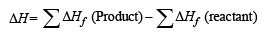Given, ΔHf CO2(g) = –393.5 kJ/mol
ΔHf CO(g) = – 110.5 kJ/mol
ΔHf H2O2(g) = – 241.8 kJ/mol

∴ ΔH = [ΔHf CO( g) +ΔHf H2O(g)]
– [ΔHf CO2(g) +ΔHf H2(g)]
= [– 110.5 + (– 241.8)] – (– 393.5 + 0)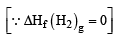= 41.2 kJ mol–1

Test: Single Correct MCQs: Thermodynamics | JEE Advanced - Question 4

In thermodynamics, a process is called reversible when (2001S)

Detailed Solution for Test: Single Correct MCQs: Thermodynamics | JEE Advanced - Question 4

In a reversible process, the driving and the opposite forces are nearly equal, hence the system and the surroundings  always remain in equilibrium with each other.

Test: Single Correct MCQs: Thermodynamics | JEE Advanced - Question 5

Which one of the following statements is false? (2001S)

Detailed Solution for Test: Single Correct MCQs: Thermodynamics | JEE Advanced - Question 5

Work is not a state function because it depends upon the path followed.

Test: Single Correct MCQs: Thermodynamics | JEE Advanced - Question 6

One mole of a non-ideal gas undergoes a change of state (2.0 atm, 3.0L, 95(K) → (4.0 atm, 5.0 L, 245K) with a change in internal energy, ΔU= 30.0L atm . The change in enthalpy (ΔH) of the process in L atm is (2002S)

Detailed Solution for Test: Single Correct MCQs: Thermodynamics | JEE Advanced - Question 6

TIPS/Formulae : ΔH =  ΔU +P2V2 – P1V1 Given, ΔU = 30.0 L atm
P1 = 2.0 atm, V1 = 3.0 L, T1 = 95 K P2 = 4.0 atm,
V2 = 5.0 L, T2 = 245 K ΔH  = ΔU + P2V2 – P1V1
= 30 + (4 × 5) – (2 × 3)  = 30 + 20 – 6 = 44 L atm.

Test: Single Correct MCQs: Thermodynamics | JEE Advanced - Question 7

Which of the reaction defines ΔH°f ? (2003S)

Detailed Solution for Test: Single Correct MCQs: Thermodynamics | JEE Advanced - Question 7

TIPS/Formulae : ΔH°f is the enthalpy change when 1 mole of the substance is formed from its elements in their standard states.
In (a) carbon is present in diamond however standard state of carbon is graphite. Again, in (d) CO (g) is involved so it can’t be the right option. Further in (c) 2 moles of NH3 are generated. Hence the correct option is (b).

Test: Single Correct MCQs: Thermodynamics | JEE Advanced - Question 8

Two moles of an ideal gas is expanded isothermally and reversibly from 1 litre to 10 litre at 300 K. The enthalpy change (in kJ) for the process is (2004S)

Detailed Solution for Test: Single Correct MCQs: Thermodynamics | JEE Advanced - Question 8

ΔH = nCp ΔT solution; since ΔT = 0 so, ΔH = 0

Test: Single Correct MCQs: Thermodynamics | JEE Advanced - Question 9

The enthalpy of vapourization of liquid is 30 kJ mol–1 and entropy of vapourization is 75 J mol–1 K. The boiling point of the liquid at 1 atm is (2004S)

Detailed Solution for Test: Single Correct MCQs: Thermodynamics | JEE Advanced - Question 9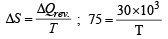∴ T = 400 K

Test: Single Correct MCQs: Thermodynamics | JEE Advanced - Question 10

The direct conversion of A to B is difficult, hence it is carried out by the following shown path :

Given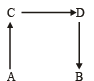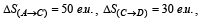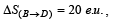where e.u. is the entropy unit, then ΔS(A→B) is (2006 - 3M, –1)

Detailed Solution for Test: Single Correct MCQs: Thermodynamics | JEE Advanced - Question 10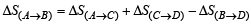Test: Single Correct MCQs: Thermodynamics | JEE Advanced - Question 11

The value of log10 K for a reaction A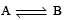B is (Given : 1 ΔrH°298K =-54.07 kJ mol-1,  ΔrS°298K = 10 JK–1 mol–1 and R = 8.314 JK–1 mol–1;  2.303 × 8.314 × 298 = 5705) (2007)

Detailed Solution for Test: Single Correct MCQs: Thermodynamics | JEE Advanced - Question 11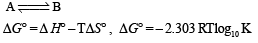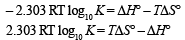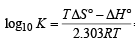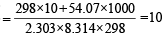Test: Single Correct MCQs: Thermodynamics | JEE Advanced - Question 12

For the process H2O(l) (1 bar, 373 K) → H2O(g) (1 bar, 373 K), the correct set of thermodynamic parameters is (2007)

Detailed Solution for Test: Single Correct MCQs: Thermodynamics | JEE Advanced - Question 12

Since, liquid is passing in to gaseous phase so entropy will increase and at 373 K during the phase transformation it remains at equilibrium. So, ΔG = 0.

Test: Single Correct MCQs: Thermodynamics | JEE Advanced - Question 13

The species which by definition has ZERO standard molar enthalpy of formation at 298 K is (2010)

Detailed Solution for Test: Single Correct MCQs: Thermodynamics | JEE Advanced - Question 13

The species in its elemental form has zero standard molar enthalpy of formation at 298 K. At 298K, Cl2 is gas while Br2 is liquid.

Test: Single Correct MCQs: Thermodynamics | JEE Advanced - Question 14

The standard enthalpies of formation of CO2(g), H2O(l) and glucose(s) at 25°C are –400 kJ/mol, –300 kJ/mol and –1300 kJ/mol, respectively. The standard enthalpy of combustion per gram of glucose at 25°C is (JEE Advanced 2013-I)

Detailed Solution for Test: Single Correct MCQs: Thermodynamics | JEE Advanced - Question 14

The standard enthalpy of the combustion of glucose can be calculated by the eqn.

C6H12O6(s) + 6O2(g) → 6CO2(g) + 6H2O(l)
ΔHC = 6 × ΔHf(CO2) + 6 × ΔHf (H2O) – ΔHf [C6H12O6]
ΔH° = 6 (–400) + 6(–300) – (–1300) = –2900 kJ/mol

For one gram of glucose, enthalpy of combustion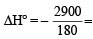- 16.11 kJ / gm

Test: Single Correct MCQs: Thermodynamics | JEE Advanced - Question 15

For the process (JEE Adv. 2014) H2O(l) → H2O(g) at T = 100°C and 1 atmosphere pressure, the correct choice is

Detailed Solution for Test: Single Correct MCQs: Thermodynamics | JEE Advanced - Question 15

Given conditions are boiling conditions for water due to which
ΔStotal = 0
ΔSsystem + ΔSsurroundings = 0
ΔSsystem = – ΔSsurroundings
For process, ΔSsystem > 0 ΔSsurroundings < 0

Test: Single Correct MCQs: Thermodynamics | JEE Advanced - Question 16

One mole of an ideal gas at 300 K in thermal contact with surroundings expands isothermally from 1.0 L to 2.0 L against a constant pressure of 3.0 atm. In this process, the change in entropy of surroundings (ΔSsurr) in JK–1 is (1 L atm = 101.3 J) (JEE Adv. 2016)

Detailed Solution for Test: Single Correct MCQs: Thermodynamics | JEE Advanced - Question 16

From 1st law of thermodynamics
qsys = ΔU – w = 0 – [–Pext.ΔV]
= 3.0 atm × (2.0 L – 1.0 L) = 3.0 L-atm

∴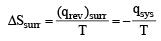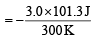= –1 .01 3 J/K

## Chemistry for JEE

298 videos|517 docs|369 tests
 Use Code STAYHOME200 and get INR 200 additional OFF Use Coupon Code
Information about Test: Single Correct MCQs: Thermodynamics | JEE Advanced Page
In this test you can find the Exam questions for Test: Single Correct MCQs: Thermodynamics | JEE Advanced solved & explained in the simplest way possible. Besides giving Questions and answers for Test: Single Correct MCQs: Thermodynamics | JEE Advanced, EduRev gives you an ample number of Online tests for practice

## Chemistry for JEE

298 videos|517 docs|369 tests